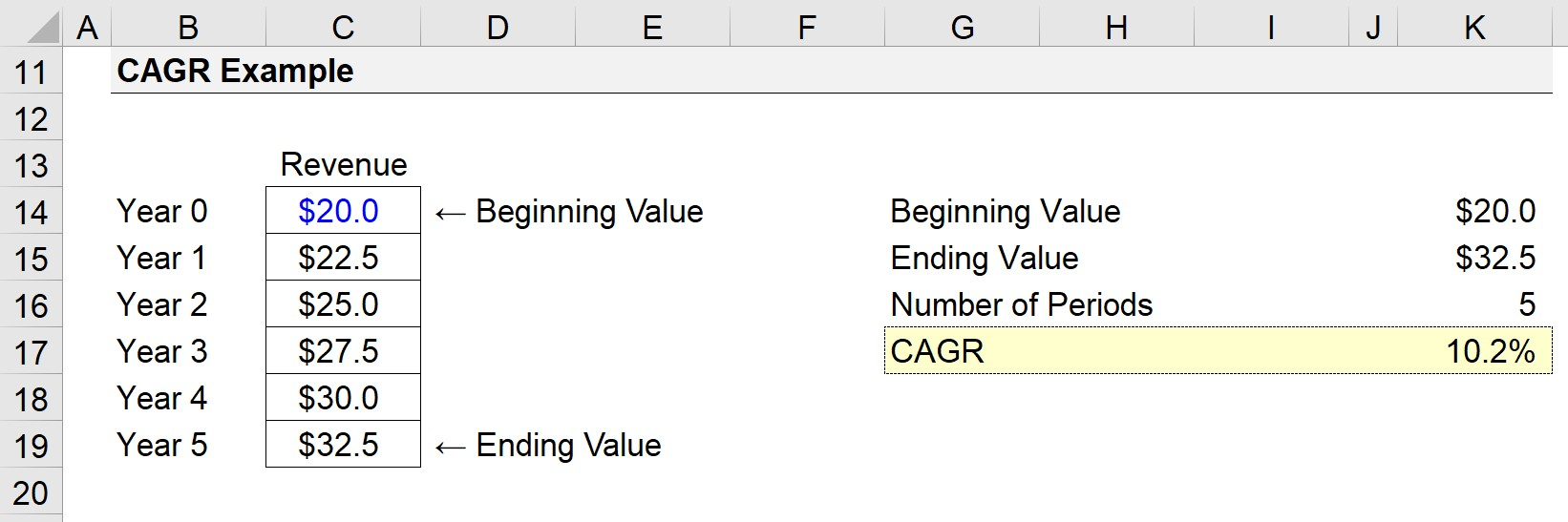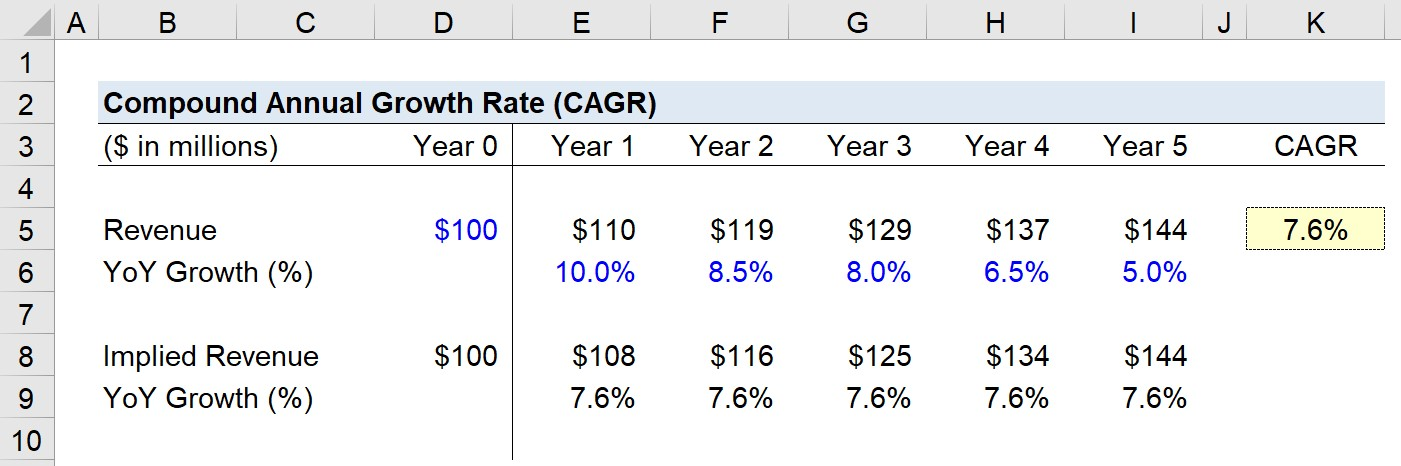# Compound Annual Growth Rate (CAGR)

Guide to Understanding the Compound Annual Growth Rate (CAGR)• CAGR stands for “Compound Annual Growth Rate”.
• CAGR is defined as the annualized growth rate in the value of a financial metric – such as revenue and EBITDA – or an investment across a specified period.
• CAGR is calculated to measure the rate of change, expressed on an annual basis, wherein the effects of compounding are taken into account.
• CAGR Formula: CAGR = (Ending Value ÷ Beginning Value) ^ (1 ÷ Number of Compounding Period) – 1

## What is the CAGR Definition?

The compound annual growth rate, or “CAGR”, is the rate of return required for the value of an investment or financial metric to grow from its beginning value to its ending value between two dates.

Conceptually, the CAGR metric answers the following question, “At what growth rate must the metric grow at each [Period] to reach [Ending Value] from [Beginning Value] under the time frame of [Number of Periods]?”

Therefore, the compound annual growth rate (CAGR) is the rate of return of an investment (or financial metric) across a predefined period, expressed in terms of an annual percentage.

But since CAGR measures growth as if the underlying investment or metric had a grown at a fixed rate while compounding on an annual basis. So, CAGR is an “estimate” of the rate of return per year – based on the pattern on growth in historical periods – rather than an actual annual historical growth rate.

## How to Calculate CAGR?

There are a total of three inputs in the CAGR formula, which are each described in the following list:

1. Beginning Value → The value at the beginning of the period (BoP).
2. Ending Value → The value at the end of the period (EoP).
3. Number of Periods (t) → The total number of compounding periods.

The CAGR calculation is a three-step process:

1. Divide the Ending Value by the Beginning Value (i.e. Initial Value)
2. Raise the Resulting Figure to the Inverse Number of Compounding Periods (1 / t)
3. Subtract One to Convert the Implied CAGR into Percent Form

## CAGR Formula

The formula for calculating the compound annual growth rate (CAGR) is as follows.

CAGR = (Ending Value ÷ Beginning Value) ^ (1 ÷ Number of Periods) 1
• Ending Value → The final value at the end of the period (EoP).
• Beginning Value → The initial value as of the beginning of the period (BoP).
• Number of Periods (t) → The total number of compounding periods.

## Quick CAGR Calculation Example

Suppose there is a company with revenue of \$20 million at the end of the current period (Year 0).

Five years from the present date, the company’s revenue is projected to reach \$32.5 million (Year 5).

Given those assumptions, we’ll enter the following figures into the CAGR formula:

• Beginning Value = \$20 million
• Ending Value = \$32.5 million
• Number of Periods = 5 Years

In the first part of the formula, the ending value of \$32.5 million is divided by the starting value of \$20 million.

The resulting figure must then be annualized by raising it to the power of 1 divided by the 5 periods.

Lastly, once we subtract 1 from the return value, we are left with a CAGR of 10.2%.

• Compound Annual Growth Rate (CAGR) = (\$32.5 million ÷ \$20.0 million)^(1 ÷ 5 Periods) – 1 = 10.2%

Note: Year 0 is excluded when counting the number of periods because only the periods when the revenue is compounding must be counted. Thus, we subtract the beginning period number from the ending period number (i.e. Year 5 minus Year 0 = 5 Years).## What is a Good CAGR?

The most notable benefit of the compound annual growth rate (CAGR) metric is the relative ease at which it can be computed, while still providing valuable insights into the growth profile of anything that rises (or falls) in value.

The financial performance of a particular company (e.g. revenue growth, EBITDA growth) or the investment performance of a portfolio can be measured using the CAGR, which reflects the versatility of the metric.

Because the CAGR can confirm whether the projections align with the industry average and historical growth, the CAGR metric can also be useful as a sanity check, i.e. to confirm the assumptions are reasonable.

Suppose a company’s revenue is projected to grow at a CAGR of 20% but the company’s closest comparables are expected to grow around 5% while the collective industry is being forecasted to grow 3% across the same periods.

## What is the Conceptual Meaning of CAGR?

Here, the company’s growth assumptions would likely warrant adjustments of some sort, or at the very least, a closer look into whether the numbers are reasonable or not.

Since annualized growth metrics remove the fluctuations of year-over-year growth rates, this helps facilitate the comparisons of CAGR over time between two companies or investments, which would otherwise be very challenging to compare.

The main drawback to the CAGR is that it fails to take into account the volatility associated with the underlying asset. Thus, the growth metric becomes vulnerable to misinterpretations, as the actual growth rate experienced year-over-year may vary.

For instance, a company’s revenue growth could in reality be disproportionate, with positive growth being front-ended in the earlier periods and ultimately tapering off or even flattening.

In the absence of understanding the contextual details of the specific scenario on hand, the CAGR can be misleading by erroneously portraying the company as having consistent positive growth potential.

## CAGR Calculator – Excel Template

We’ll now move on to a modeling exercise, which you can access by filling out the form below.Submitting ...

## 1. Revenue Growth Assumptions

Suppose we are tasked with calculating the compound annual growth rate (CAGR) of a company’s revenue.

At the end of the current period, the company has generated \$100 million in revenue – and this figure is anticipated to grow at the following growth rates each year.

• Year 0 → Year 1: 10.0%
• Year 1 → Year 2: 8.5%
• Year 2 → Year 3: 8.0%
• Year 3 → Year 4: 6.5%
• Year 4 → Year 5: 5.0%

By the end of Year 5, the company’s revenue reaches \$144 million, after starting at \$100 million in Year 0.

## 2. CAGR Calculation Example

Like the previous example, we are going to input the following assumptions into the CAGR formula:

• Beginning Value = \$100 million
• Ending Value: \$144 million
• Number of Periods: 5 Years

Our CAGR formula divides \$144 million (i.e. the future value) by \$100 million (i.e. the present value) and then raises it to 1 divided by 5 (i.e. the number of periods).

In the subsequent step, we subtract by 1 to get 7.6% as the implied CAGR.

• Compound Annual Growth Rate = (\$144 million / \$100 million)^(1 / 5 Periods) – 1
• CAGR = 7.6%

The row below the revenue figures calculates the year-over-year growth rates of revenue.

An alternative method to determine the historical or expected CAGR is to utilize the “RATE” function in Excel.

The COUNTA function can count the number of years (nper) for the first input, and we can hard-code a zero for the next input (pmt).

In the next steps, we can enter -\$100 million for the present value (pv), and \$144 million for the future value (fv), with a negative sign placed in front of either 1) the present value or 2) the future value.

## 3. CAGR Analysis

Contrary to a common misconception, the calculation of CAGR is not as simple as averaging the YoY growth rates.

But rather, given the initial value, ending value and specific date parameters, the CAGR metric assumes the profits are reinvested each period and that interest is compounded annually.

To ensure that you understand the concept of CAGR, we have also computed the implied revenue, which we link to the \$100 million assumption for Year 0 and then grow it by the CAGR of 7.6%.

At the end of Year 5, we can see how by growing revenue by the constant rate of 7.6% each year, the company’s revenue reaches \$144 million, confirming our calculation of CAGR was correct.

Annualized metrics such as the CAGR metric should not be taken at face value to minimize the risk of understating (or overstating) the growth potential without understanding the volatility risks and core drivers of performance.

In closing, the compound annual growth rate (CAGR) is a practical metric to facilitate sound decision-making, yet it is critical to understand that the CAGR as a standalone metric still does not reveal the entire story.Step-by-Step Online Course

### Everything You Need To Master Financial Modeling

Enroll in The Premium Package: Learn Financial Statement Modeling, DCF, M&A, LBO and Comps. The same training program used at top investment banks.Inline FeedbacksLearn Financial Modeling Online

Everything you need to master financial and valuation modeling: 3-Statement Modeling, DCF, Comps, M&A and LBO.

X

The Wall Street Prep Quicklesson Series

7 Free Financial Modeling Lessons

Get instant access to video lessons taught by experienced investment bankers. Learn financial statement modeling, DCF, M&A, LBO, Comps and Excel shortcuts.